## KCSE 2015 Mathematics Alt A Paper 1 with Marking Scheme

SECTION I (50 marks)
Answer all questions in this section in the spaces provided.

1.
1. Evaluate 540 396 - 726450 ÷ 3 (1 mark)
2. Write the total value of the digit in the thousands place of the results obtained in (a) above.  (1 mark)
2. Muya had a 6⅔ ha piece of land. He donated ⅞ ha to a school and 1½ ha to a childrens home. The rest of the land was shared equally between the son and the daughter. Find the size of the land that each child got. (3 marks)
3. The volume of a cube is 1 728 cm3. Calculate, correct to two decimal places, the length of the diagonal face of the cube.    (3 marks)
4. Use logarithms correct to 4 significant figures, to evaluate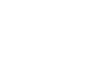(4 marks)
5. A piece of wire is bent into the shape of an isosceles triangle. The base angles are each 48o and the perpendicular height of the base is 6 cm. Calculate, correct to one decimal place, the length of the wire.  (3 marks)
6. The density of a substance A is given as 13.6 g/cm3 and that of a substance B as 11.3 g/cm3. Determine, correct to one decimal place, the volume of B that would have the same mass as 50 cm3 of A.  (3 marks)
7. Below is a part of a sketch of a solid cuboid ABCDEFGH. Complete the sketch.  (2 marks)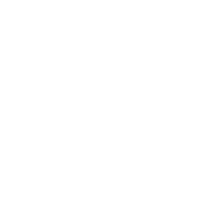8. A salesman is paid a salary of Kshs 15,375 per month. He also gets a commission of 4½ % on the amount of money he makes from his sales. In a certain month he earned a total of Ksh 28,875. Calculate the value of his sales that month. (3 marks)
9. The sum of interior angles of a regular polygon is 24 times the size of an exterior angle.
1. Find the number of sides of the polygon. (3 marks)
2. Name the polygon (1 mark)
10. The marks scored by a group of students in a test were recorded in a table as shown below.
 Marks 30-34 35-39 40-44 45-49 50-54 55-59 60-64 No. of Students 3 6 5 12 8 9 7
On the grid provided, and on the same axes, represent the above data using:
1. a histogram (3 marks)
2. a frequency polygon (1mark)
11. Given that P = 5a -2b where a =(32) and b = (41). Find:
1. column vector P; (2 marks)
2. P', the image of P under a translation vector (-64) (1 mark)
12. Given that a = 3, b= 5 and c = -½, evaluate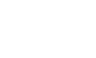(3 marks)
13. The figure below represents the curve of an equation.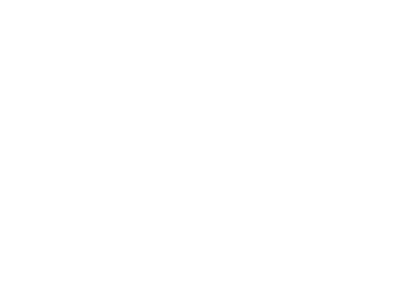Use the mid-ordinate rule with 4 ordinates to estimate the area bounded by the curve, lines y = 0, x= -3 and x = 5. (3 marks)
14. The cost of 2 jackets and 3 shirts was Ksh 1,800. After the cost of a jacket and that of a shirt was increased 20%, the cost of 6 jackets and 2 shirts was Ksh 4,800. Calculate the new cost of a jacket and that of a shirt. (4 marks)
15. A tailor had a piece of cloth in the shape of a trapezium. The perpendicular distance between the two parallel edges was 30 cm. The length of two parallel edges were 36 cm and 60 cm. The tailor cut off a semi circular piece of the cloth of radius 14 cm from the 60 cm edge. Calculate the area of the remaining piece of cloth. (Take π = 22/7) (3 marks)
16. Musa cycled from his home to a school 6 km away in 20 minutes. He stopped at the school for 5 minutes before taking a motorbike to a town 40 km away. The motorbike travelled at 75km/h. On the grid provided draw a distance-time graph to represent Musa's journey. (3 marks)

SECTION II (50 marks)
Answer any five questions in this section in the spaces provided.

1. Three partners Amina, Bosire and Karuri contributed a total of Ksh 4,800,000 in the ratio 4:5:7 to buy an 8 hectares piece of land. The partners set aside ¼ of the land for social amenities and sub-divided the ret into 15 m by 25 m plots.
1. Find
1. The amount of money contributed by Karuri. (2 marks)
2. The number of plots that were obtained. (3 marks)
2. The partners sold the land at Ksh 50 000 each and spent 30% of the profit realised to pay for administrative costs. They shared the rest of the profit in the ratio of their contributions.
1. Calculate the net profit realised. (3 marks)
2. Find the difference in the amount of the profit earned by Amina and Bosire. (2 marks)
2. Two shopkeepers, Juma and Wanjiku bought some items from a wholesaler. Juma bought 18 loaves of bread, 40 packets of milk and 5 bars of soap while Wanjiku bought 15 loaves of bread, 30 packets of milk and 6 bars of soap. The prices of a loaf of bread, a packet of milk and a bar of soap were Ksh 45, Ksh 50 and Ksh 150 respectively.
1. Represent
1. The number of items bought by Juma and Wanjiku using a 2 x 3 matrix. (1 mark)
2. The prices of the items bought using a 3 x 1 matrix. (1 mark)
2. Use the matrices in (a) above to determine the total expenditure incurred by each person and hence the difference in their expenditure. (3 marks)
3. Wanjiku and Juma also bought rice and sugar. Juma bought 36 kg of rice and 23 kg of sugar and paid Ksh 8,160. Wanjiku bought 50 kg of rice and 32 kg of sugar and paid Ksh 11,340. Use the matrix method to determine the the price of one kilogram of rice and one kilogram of sugar. (5 marks)
3. Line AB below is a side of a triangle ABC.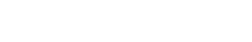1. Using a pair of compasses and a ruler only construct:
1. Triangle ABC in which BC = 10cm and <CAB = 900; (2 marks)
2. A rhombus BCDE such that <CBE = 1200; (2 marks)
3. A perpendicular from F, the point of intersections of the diagonals of the rhombus, to meet BE at G. Measure FG; (2 marks)
4. A circle to touch all the sides of the rhombus. (1 mark)
2. Determine the area of the region in the rhombus that lies in the outside of the circle. (3 marks)
4. In the figure below, AC = 12 cm, AD = 15 cm and B is point on AC. <BAD = <ADB = 300.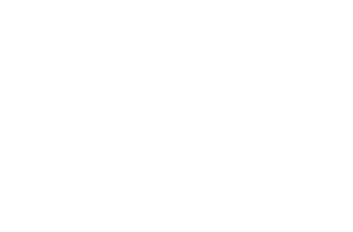Calculate, correct to one decimal place:
1. The length of CD; (3 marks)
2. The length of AB; (3 marks)
3. The area of triangle BCD; (2 marks)
4. The size of < BCD. (2 marks)
5.
1. A straight line L1 whose equation is 3y-2x = -2 meets the x axis at R. Determine the co-ordinates of R. (2 marks)
2. A second line L2 is perpendicular to L1 at R. Find the equation L2 in the form y = mx+ c, where m and c are constants. (3 marks)
3. A third line L2 passes through (-4, 1) and is parallel to line L1. Find:
1. The equation of L3 in the form of y = mx+c, where m and c are constants. (2 marks)
2. The co-ordinates of point S, at which L3 intersects L2. (3 marks)
6. On the grid below, an object T and its image T' are drawn.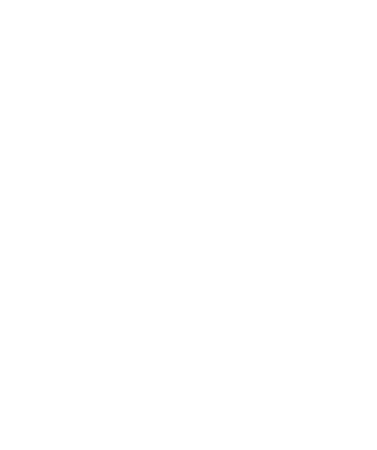1. Find the equation of the mirror line that maps T onto T' (1 mark)
2.
1. T' is mapped onto T'' by positive quarter turn about (0,0). Draw T''. (2 marks)
2. Describe a single transformation that maps T onto T''. (2 marks)
3. T'' is mapped onto T''' by an enlargement, centre (2,0), scale factor -2. Draw T'''. (2 marks)
4. Given that the area of T''' is 12 cm2, calculate the area of T. (3 marks)
7. The figure below represents a conical flask. The flask consists of a cylindrical part and a frustum of a cone. The diameter of the base is 10 cm while that of the neck is 2 cm. The vertical height of the flask is 12 cm.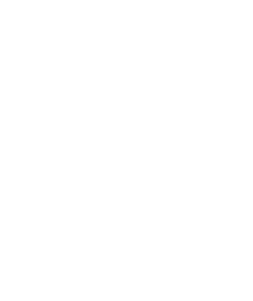Calculate, correct to 1 decimal place :
1. The slant height of the frustum part; (2 marks)
2. the slant height of the smaller cone that was cut off to make the frustum part. (2 marks)
3. The external surface area of the flask. (Take π = 3.142 ) (6 marks)
8. The gradient of the curve y = 2x- 9x+ px - 1 at x = 4 is 36.
1. Find:
1. The value of p; (3 marks)
2. The equation to the tangent of the curve at x = 0.5. (4 marks)
2. Find the co-ordinates of the turning points of the curve. (3 marks)## MARKING SCHEME

1.
1. 540396 - 726450 - 3
= 540396 - 242150 = 298246
2. Total value of digit in the thousands place
= 8000
2. 6 2/3 -(7/8 + 1½)
103
24
Son/daughters share = ½ x 103
24
= 27/48 ha
3. S= 3√1728 = 12
let the diagonal bed cm
d = √122 +122
= 16.97

4.  No - Log 72.56 0.64 1.8607 1.8062 or -0.1938 1.6669 1.845 0.2660 x      2 0.53201.6669 0.5320 1.1349 ÷ 2 3.694 0.5675
M1 logs
MI  division and multiplication
5.  ½a  = tan 42º
6
a = 12 tan 42º
= 10.8
6    = c or b
sin 48
= 8.07
length = 10.8 + 2 x 8.07
= 26.9 cm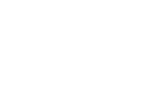6. Mass of A= 50 x 13.6
= 680 g
Volume of B = 680
11.3
= 60.2 cm3

7.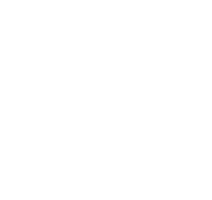B1 transfer of all lines ✓
B1 hidden lines and labelling of cuboid
8. Commission amount = 28875 - 15375
= 13500
4.5  x= 13500
100
= 13500 x 100
4.5
= 300 000
9.
1. (2n - 4) 90º = 360 x 24
n
n2 - 2n - 48 = 0
(n-8)(n+6)=0
n=8
2. Octagon

10.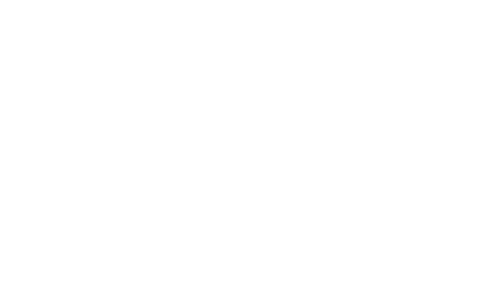S1 scale
B2 All bars V (B1 for 4 - 6 bars)
B1 frequency polygon

11.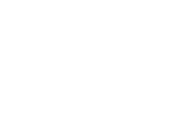M1
A1
B1
12. 4a2 +2b-4c = 4 x 32 +2 x 5 -4 x -1/2
¼(b - 3a)         ¼(52 - 3 x 3)
= 4 x 9 +10 + 2
¼(25 - 9)
48    =12
4
13. Area under the curve:
Ordinates: 6,2, 6 and 18
area = 2 (6 +2 + 6+ 18)
= 64
14. 2j+ 38 = 1800
7.2j +2.4s = 4800
7.2j + 10.8s = 6480
7.2j +2.4s = 4800
8.4s = 1680
S = 200
j=600
New costs: Shirt - 200 X 1.2 = 240
jacket - 600 x 1.2 = 720
B1 for both new costs
M1 Formation of the 2 simultenous equations
M1 Attempt to solve for j or s
A1 for both values
15. Trapezium:
½ x 30 x (36 +60)
= 1440
Semi circle: ½ x 22/7 x 14 x 14
= 308
Remaining part:
1440 - 308 = 1132 cm2
16. Time of motorbike
= 40 x 60 = 32 min.
75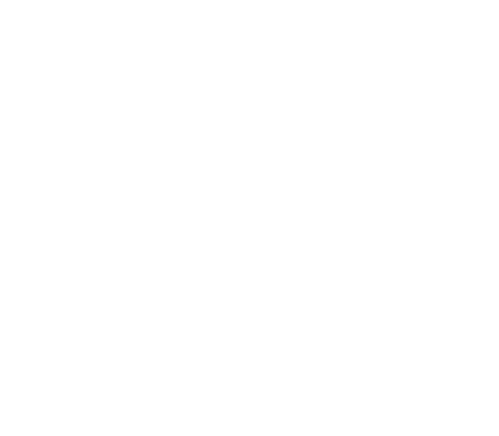Graph for cycling and stopping
Graph for motorbike
17.
1.
1. sh  7   x 4800 000
16
= 2100000
2. 3/4 x 8 x 10000 m2
15 x 25
= 160
MI Conversion
MI Division by Area
2.
1. Profit
50 000 x 160 - 4 800 000
= 3 200 000
Net profit = 70  x 3 200 000
100
= 2 240 000
2. Amount earned by A and B
=5 - 4 x 2 240 000
16
= 140 000
18.
1.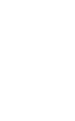2.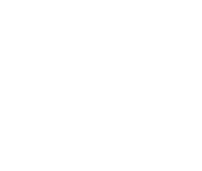Difference = 3560 - 3075 = 485
3. Let price of rice be x and that of sugar by y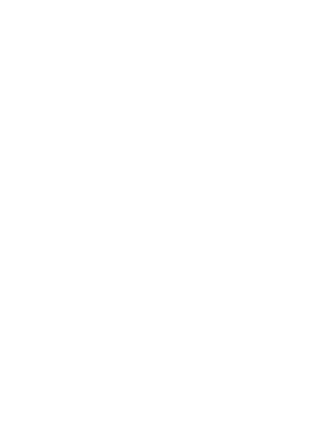19.

1.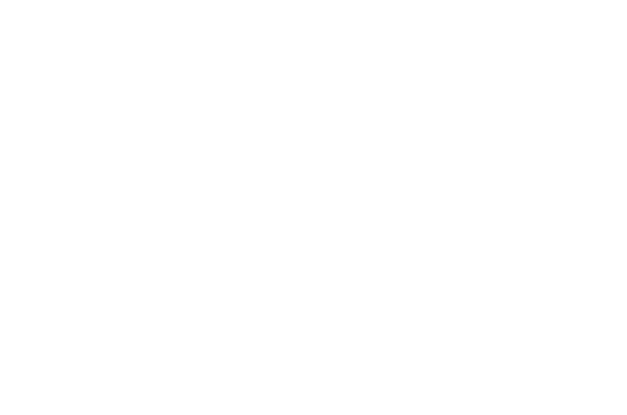1. Construction of 90° at A
completion of triangle ABC
2. Construction of 120°
completion of rhombus
3. Dropping perpendicular from F to BE
length of perpendicular = 4.3 +0.1 cm
4. Construction of circle touching sides of rhombus
B1 circle constructed
2. area of rhombus outside the circle
2(½ x 10 x 10 sin60) - π x 4.32
86.6 - 58.1
= 28.5 cm2
M1 area of rhombus area of circle
M Subtraction
20.
1. CD2 = 122 + 152 - 2 x 12 x 15 cos30°
= 144 +225 - 311.8
= 57.2
CD = √57.2 = 7.6 cm
2.    AB   =    15
sin 30    sin 120
AB =15 sin30
sin120
= 8.7 cm
3. DBC = 60°, BC = 12 -8.7 = 3.3
and BD = 8.7
area = ½ x 3.3 x 8.7 sin 60
= 12.4 cm2
4. angle BDC:
3.3   =   7.6   sinθ = 3.3 sin 60
sinθ      sin 60                7.6
θ = 22.1°
21.
1. At x-axis y = 0   x=1
coordinates of Ris (1,0)
Equation of L2 y - 0 = -3
x - 1    2
y = 3/2 x + 1½
3.
1. Equation L3; y - 1  =
x + 4     3
3y - 3 = 2x + 8
y = 2/3x + 32/3
2. 2/3x + 32/-3 x +
2       2
subst for y: y=2/(-1)+ 11/3
= -2 + 11  = 3
3       3
coordinates of S (-1,3)
22.
1. mirror line - y = -x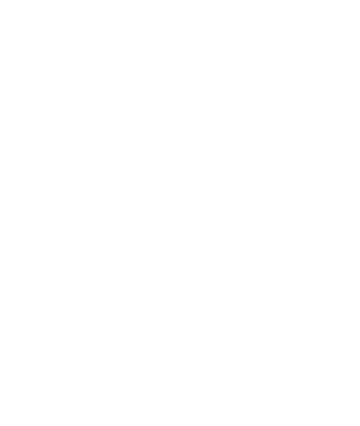2.
1. points A, B and Co
drawing A'B' C'
2. reflection
on line y = 0
points A, B and Co drawing A'B' C'
3.  points A, B and C drawing A'B' C'
4. area scale factor (-2)2 = 4
area of object = 12
4
= 3 cm2
23.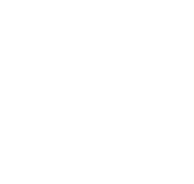1. slant height = √42 +102
= 10.8
2. slant height of small cone (1)
l l +10.8
1         5
5l = l + 10.8     l = 2.7
3. circular bottom: 3.142 x 52 = 78.6
cylindrical neck: 3.142 x 2 x 2 = 12.6
conical part:
3.142 x 5 x 13.5 - 3.142 x 1 x 2.7
212.1-8.5 = 203.6
External surface area = 78.6 + 12.6 + 203.6
= 294.8
24.
1.
1.  dy  = 6x2 - 18x+p
dx
at x = 4: dy = 6 x 16 - 18 x 4+ p = 36
dx
96 - 72 + p = 36
p=12
2. At x = 0.5
dy  = 6 x (0.5)2 - 18 x 0.5 +12
dx
= 4.5
At x=0.5
y = 2(0.5)3 - 9 x 0.52 + 12 x 0.5 - 1
Equation = y - 3 =
x - ½    2
9(x -½) =2(y-3)
9x - 9/2 = 2y - 6
2y - 9x =3/2
4y - 18x = 3
2. At turning point dy = 0
dx
i.e  6x2 - 18x + 12 = 0
(x - 1)(x - 2) = 0
x = 1 or x = 2
coordinates (1,4)
(2,3)

#### Download KCSE 2015 Mathematics Alt A Paper 1 with Marking Scheme.

• ✔ To read offline at any time.
• ✔ To Print at your convenience
• ✔ Share Easily with Friends / Students